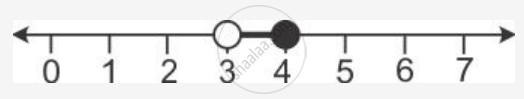Share

# Solve the Inequation: 3z – 5 ≤ Z + 3 < 5z – 9, Z ∈ R. Graph the Solution Set on the Number Line - Mathematics

Course

#### Question

Solve the inequation:
3z – 5 ≤ z + 3 < 5z – 9, z ∈ R.
Graph the solution set on the number line

#### Solution

3z - 5  ≤ z + 3 < 5z - 9

3z - 5  ≤ z + 3 and z + 3 < 5z - 9

2z ≤ 8 and 12 < 4z

z ≤ 4 and 3 < z

Since, z ∈ R

∴ Solution set = {3 < z ≤ 4, x ∈ R }

It can be represented on a number line as:Is there an error in this question or solution?